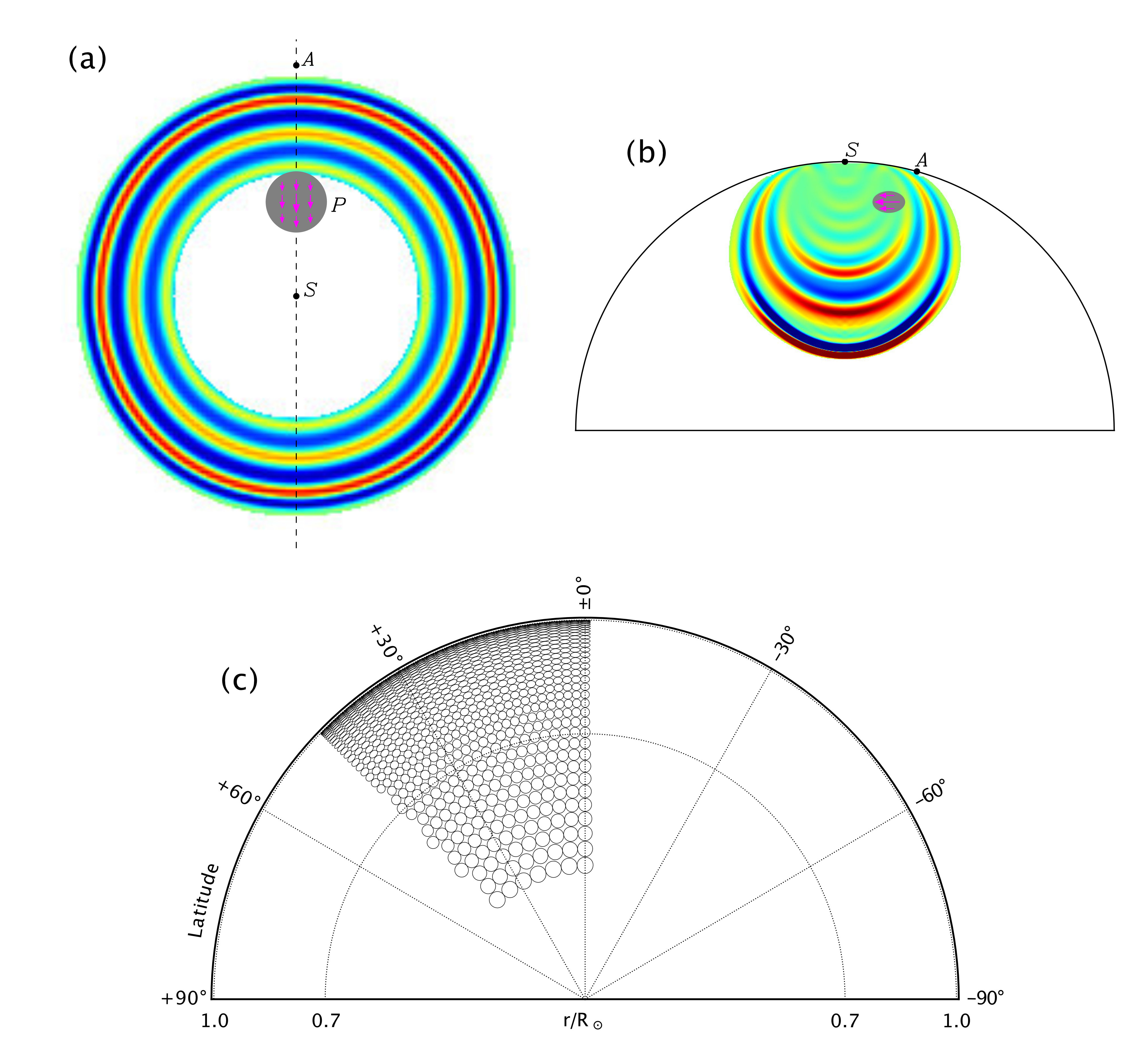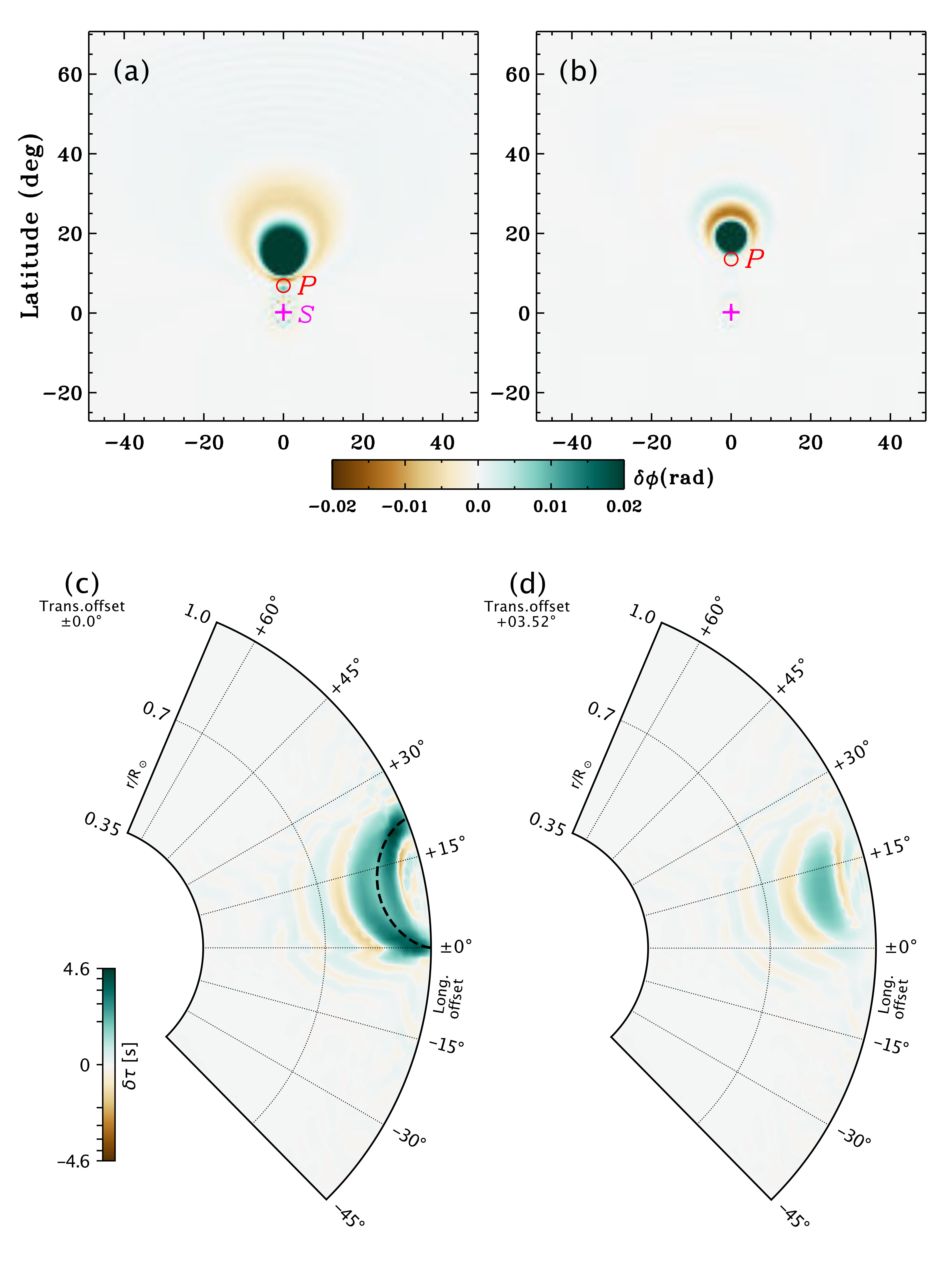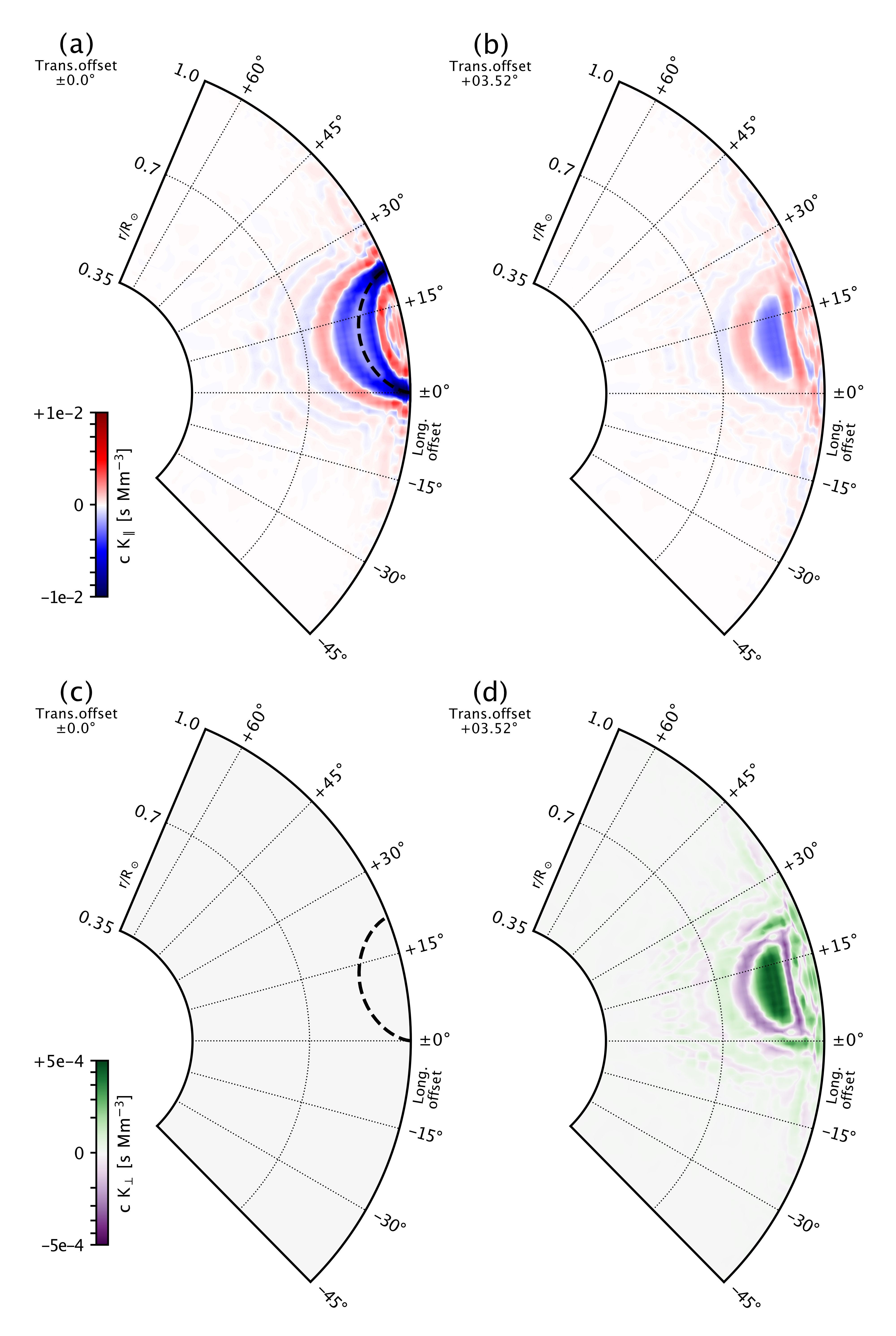# 153. Computing Helioseismic Sensitivity Kernels for the Sun’s Large-scale Internal Flows Using Global-scale Wave-propagation Simulations

Contributed by Junwei Zhao. Posted on March 28, 2021

Thomas Hartlep1,2 & Junwei Zhao3

1 Bay Area Environmental Research Institute, Moffett Field, CA 94035, USA;
2 NASA Ames Research Center, Moffett Field, CA 94035, USA
3 W. W. Hansen Experimental Physics Laboratory, Stanford University, Stanford, CA 94305-4085, USA

Time–distance helioseismology measures travel times of acoustic waves traveling between two different locations on the solar surface. To infer the Sun’s internal flows, one needs sensitivity kernels that relate the Sun’s internal flows with these surface travel-time measurements. Sensitivity kernels for internal flows have already been derived using ray approximation, Born approximation, or other methods. Here we introduce a new method to derive the sensitivity kernels for internal flows based on global-scale wavefield simulations.

The flow sensitivity kernel at a location (r, θ, φ) in the solar interior can be interpreted as the amount of travel-time (or phase) shift observable on the surface between waves traveling in opposite directions caused by an infinitesimally small flow perturbation at that location, normalized by the perturbation volume and its flow speed. By simulating wave propagation through infinitesimally small perturbations at many different locations and measuring their effect on travel times, the entire kernel could be mapped out directly. In practice, we cannot simulate infinitesimally small perturbations, and the closest we can get to this scenario is by using flow perturbations that are small relative to the finite wavelength of helioseismic waves, and with speeds that are small compared to the local sound speed to maintain linearity. Figure 1 shows the set-up of our wavefield simulation with the flow perturbations placed inside the model.Figure 1| An example of top view (a) and cross-sectional view (b) of the simulated wavefield (blue and red) with an internal flow-perturbation volume, represented by the gray area with magenta arrows indicating the flow direction. Panel (c) shows the cross-sectional view of different locations (depth and latitudinal offset from the wave source) of 784 Gaussian-shaped flow perturbations used in our wave-propagation simulations. Each simulation contains two such perturbations on opposite sides on the Sun.

All the wavefields, a total of 784 with flow perturbations and one reference model without any internal perturbation, are simulated using the “aSHBury” code. To measure surface travel-time shifts δτ, as a function of oscillatory frequency ν, the time series at each location in the selected regions are compared with the time series at the same location but taken from the reference simulation. The top panels of Figure 2 show the travel-time shifts measured in the photosphere at ν=3 mHz for two selected examples; the bottom panels show the travel-time shifts, measured at the same frequency but for a measurement distance of 21.6°, plotted in a way with the surface-measured travel-time shifts placed at the corresponding flow-perturbation locations, with some data interpolations applied.Figure 2| (Top row) Select maps of travel-time shifts for ν = 3.0 mHz for internal flow perturbations located at a depth of 54.5 Mm and distances of (a) 6.62°, (b) 13.24° away from the wave source. (Bottom row) Travel-time shifts in kernel coordinates obtained by remapping and combining surface measurement for all perturbations and all locations with a distance of d = 21.6° from the source, at a frequency of 3 mHz, at (c) 0.0° and (d) 3.52° offset from the source-receiver plane.

The equation that relates interior flows and sensitivity kernels to the surface measurements can be discretized as:$\delta\tau_i=\Sigma_j [K_j^{\parallel} u_{(i,j)}^{\parallel} + K_j^{\perp} u_{(i,j)}^{\perp}] V_j$
where$K_j^{\parallel}$ and$K_j^{\perp}$ are the unknown components of the kernel in the direction along the source-receiver line and perpendicular to it, and$u_{(i,j)}^{\parallel}$ and$u_{(i,j)}^{\perp}$ are the two known components of the perturbation velocity, also discretized in kernel coordinates. The$V_j$ are the volume elements of the discrete grid. We then solve the linear equations for the sensitivity kernels$K$. Figure 3 shows our solutions for a selected example.Figure 3| Sensitivity kernels (multiplied with sound speed c) for longitudinal flows (upper row) and transverse flows (lower row) for a measurement distance of 21.6° and a frequency of ν = 3 mHz. The different panels in each row show vertical slices of the kernel at transverse offsets from the central plane of 0.0° and 3.52° (from left to right), respectively. The dashed lines in panels (a) and (c) represent the ray path connecting the two surface locations between which the travel-time shifts are measured.

Although the examples shown throughout this text are only for ν = 3 mHz, our measurements actually yield results for all frequencies between about 2.0 and 6.0 mHz, providing rich information that can be used for our future studies of frequency-dependent time-distance helioseismic measurements. These kernels, when applied on measurements from observations, are believed to give more accurate inversions of the Sun’s large-scale internal flows.

For more details of this work, please refer to our recent publication Ref. .

### References

 Giles, P. M. 1999, Ph.D. Dissertation, Stanford Univ.
 Böning, V. G. A., Roth, M., Zima, W., Birch, A. C., & Gizon, L. 2016, ApJ, 824, 49
 Hartlep, T., Zhao, J., Kosovichev, A. G., & Mansour, N. N. 2013, ApJ, 762, 132
 Hartlep, T., & Zhao, J. 2021, ApJ, 909, 66

## One comment on “Computing Helioseismic Sensitivity Kernels for the Sun’s Large-scale Internal Flows Using Global-scale Wave-propagation Simulations”

1. Junwei Zhao

Looking forward to seeing the meridional circulation inverted from these new kernels.